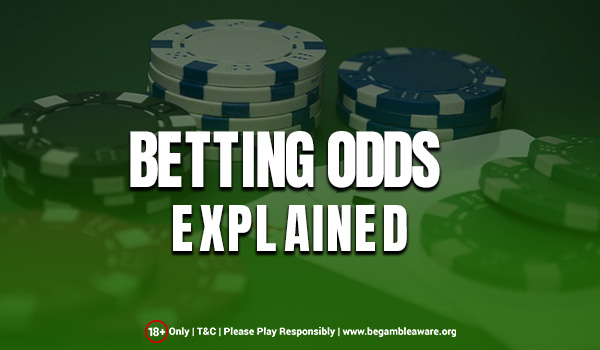# Betting Odds Explained

One of the first steps in the world of betting is to have a clear understanding of how Betting Odds work. Betting Odds are a combination of the probability around an event, that is, how likely the event will be happening and the potential winnings associated with it, which means how much can you expect to win if a particular outcome gets played out.To the beginner, all the numbers and numerous calculations may seem complex and intimidating at first, but after clear explanation and a reasonable familiarity, players will be able to appreciate the inherent logic and simplicity associated with Betting Odds.

## Definition of Probability

At the most basic and elementary level, betting gives you the ability to predict the outcome of any event that is about to happen. As an example, take an event called rolling a dice. This has a certain number of outcomes, six to be exact. For every single outcome of the dice, rolling a ‘one’, ‘two’, ‘three’, ‘four’, ‘five’, or ‘six’, there is a 16.67% chance or probability that it will happen. Betting Odds are a representation of the chances of a particular event happening. In the gambling world, these are represented in the form of a fraction, like in this case, it would be 5/1.

## Convert Odds to Probability

The odds in the betting world are represented in the form of fractions. These fractional odds are written in the form of two numbers separated by a trailing slash. As an example, 3/1. Anyone can calculate the chance of an event’s occurrence, i.e, the probability, using these betting odds. If the odds are represented as A/B the probability is calculated using the following formula:

Probability (P in %) = B/(A+B), which is B divided by the sum of A and B.

Looking at numerical examples:

• 3/1 is computed as P = 1/(3+1) = 0.25, there is a 25% probability of the event occurring
• 1/4 is computed as P = 4/(4+1) = 0.80, there is an 80% probability of the event occurring
• 1/1 is computed as P = 1/(1+1) = 0.50, there is an 50% probability of the event occurring

## Convert Betting Odds to Winnings

Odds can also be used to calculate the potential winnings if the bet is made by the player. To calculate the winnings, for odds A/B, the winnings are A for each value of B bet by the player, in addition to getting back the original stake,i.e, B.

As examples:

• 3/1 stipulates that for every bet of £1, the player wins £3
• 5/1 stipulates that for every bet of £1, the player wins £5
• 1/4 stipulates that for every bet of £4, the player wins £1

## Decimal Odds

The betting industry has slowly shifted to displaying odds in decimal form. This is due to the easy calculation of winnings and the inclusion of the returned stake in the calculation of payouts using decimal odds.

The calculation goes like this: Winnings = (odds*stake) – stake

Considering a few numerical examples:

• 9.0 is computed as (9.0 * £10 stake) – £10 stake  = £80 winnings.
• 4.0 is computed as (4.0 * £10 stake) – £10 stake  = £30 winnings
• 2.5 is computed as (2.5 * £10 stake) – £10 stake  = £15 winnings

## Display of Betting Odds

In the UK and other countries, the historical trend has been to show fractional odds for the display on racetracks or the financial street. However, recent trends point to the scales shifting in favour of decimal odds due to its simplicity and ease of use. Betfair is one of the betting exchanges that has pioneered the use of decimal odds.

Rate this post

### Blog Summary

Blog Name: Betting Odds Explained

Posted On: 28/06/2019

Author: Robert Bowron

Rate this post